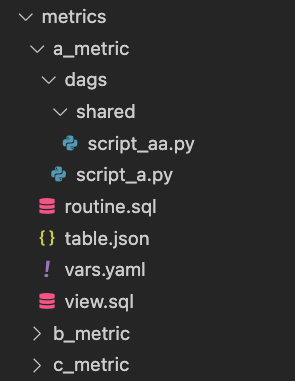# How to upload multiple files to a GCP bucket? for_each

I need to upload the files inside the dags folder of each metric to a bucket in GCP, I was able to just iterate through the folder name, what I need is the files and subfolders/files.This is my directory structure:

``````metrics/
a_metric/
dags/
shared/files
files
b_metric/
dags/
shared/files
files
c_metric/
dags/
shared/files
files
``````

main.tf:

``````locals {
# Base variables
base_vars = yamldecode(file("base/vars.yaml"))

#OK
# Metrics subfolder names
metrics = distinct([
for dir_path in fileset("metrics/", "*/*/") :
basename(dirname(dir_path))
])

# Metrics configuration
metrics_config = flatten([
for subfolder in local.metrics :
[
for file in fileset("metrics/\${subfolder}", "vars.yaml") :
merge(yamldecode(file("metrics/\${subfolder}/\${file}")), { subfolder = subfolder })
]
])
}

for_each = { for metric in local.metrics_config: metric.subfolder => metric }
name   = "dags/\${each.value.subfolder}/"
source = "metrics/\${each.value.subfolder}/dags/"
bucket = "bucket_test_dags_terraform_001"
}

``````

Plan Output:

``````Terraform will perform the following actions:

+ bucket         = "bucket_test_dags_terraform_001"
+ content_type   = (known after apply)
+ crc32c         = (known after apply)
+ detect_md5hash = "different hash"
+ id             = (known after apply)
+ kms_key_name   = (known after apply)
+ md5hash        = (known after apply)
+ media_link     = (known after apply)
+ name           = "dags/a_metric/"
+ output_name    = (known after apply)
+ self_link      = (known after apply)
+ source         = "metrics/a_metric/dags/"
+ storage_class  = (known after apply)
}

+ bucket         = "bucket_test_dags_terraform_001"
+ content_type   = (known after apply)
+ crc32c         = (known after apply)
+ detect_md5hash = "different hash"
+ id             = (known after apply)
+ kms_key_name   = (known after apply)
+ md5hash        = (known after apply)
+ media_link     = (known after apply)
+ name           = "dags/b_metric/"
+ output_name    = (known after apply)
+ self_link      = (known after apply)
+ source         = "metrics/b_metric/dags/"
+ storage_class  = (known after apply)
}

+ bucket         = "bucket_test_dags_terraform_001"
+ content_type   = (known after apply)
+ crc32c         = (known after apply)
+ detect_md5hash = "different hash"
+ id             = (known after apply)
+ kms_key_name   = (known after apply)
+ md5hash        = (known after apply)
+ media_link     = (known after apply)
+ name           = "dags/c_metric/"
+ output_name    = (known after apply)
+ self_link      = (known after apply)
+ source         = "metrics/c_metric/dags/"
+ storage_class  = (known after apply)
}

Plan: 3 to add, 0 to change, 0 to destroy.
``````